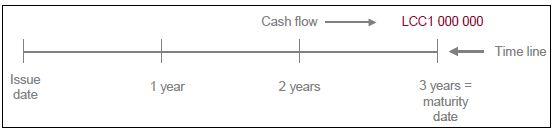Finance

# Explain Zero Coupon Bonds versus Coupon Bonds

A zero coupon bond, as the name indicates, is a bond that does not have any coupon payments. An example follows (Figure):

Nominal value:            LCC 1000 000

Term:                           3 years

Interest payable:          None (except that the “discount” is payable)

Interest dates:             None (except that the discount amount is payable on maturity date).

The zero coupon bond contrasts with the coupon bond, and is the most straightforward of all bonds. The face of the certificate has the name of the investor, the nominal (face) value and the maturity date. It is issued at a discount rate, which reflects the interest rate that the investor is prepared to pay, i.e. the market rate for 3-year money. The return to the investor is the difference between that nominal value and the price paid, i.e. the discount amount.Figure: example of zero coupon bond